# Nowpaper 十五英寸的世界

Rich Games Developer

 博客园  :: 首页  :: 新随笔  :: 联系 :: 订阅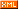:: 管理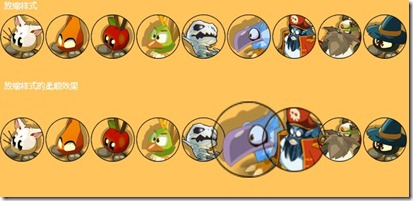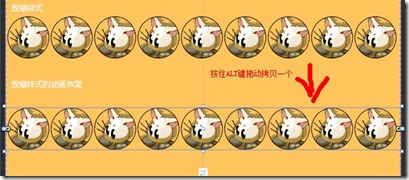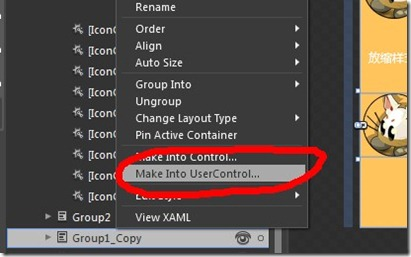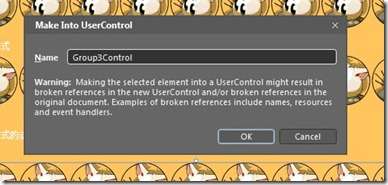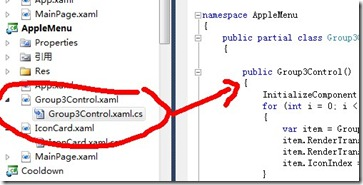Code Snippet
1. public Group3Control()
2. {
3.     InitializeComponent();
4.     for (int i = 0; i < Group3.Children.Count; i++)
5.     {
6.         var item = Group3.Children[i] as IconCard;
7.         item.RenderTransformOrigin = new Point(0.5, 0.5);
8.         item.RenderTransform = new ScaleTransform() { ScaleX = 1, ScaleY = 1 };
9.         item.IconIndex = i;
10.     }
11. }

Code Snippet
1. protected override void OnMouseMove(MouseEventArgs e)
2. {
3.
4.     foreach (var item in Group3.Children)
5.     {
6.         var t = item as IconCard;
7.         if (t == null)
8.             continue;
9.         double x = e.GetPosition(t).X - t.ActualWidth / 2;
10.         double y = e.GetPosition(t).Y - t.ActualHeight / 2;
11.         double mine = Math.Sqrt(x * x + y * y);
12.         double mineZoom = Zoom(1.2, mine, 72);
13.         (t.RenderTransform as ScaleTransform).ScaleX = mineZoom;
14.         (t.RenderTransform as ScaleTransform).ScaleY = mineZoom;
15.     }
16.     base.OnMouseLeave(e);
17. }
18. protected override void OnMouseLeave(MouseEventArgs e)
19. {
20.     foreach (var item in Group3.Children)
21.     {
22.         var t = item as IconCard;
23.         if (t == null)
24.             continue;
25.         (t.RenderTransform as ScaleTransform).ScaleX = 1;
26.         (t.RenderTransform as ScaleTransform).ScaleY = 1;
27.     }
28.
29.     base.OnMouseLeave(e);
30. }
31. double Zoom(double m, double d, double s)
32. {
33.     if (d < s)
34.     {
35.         return (m * (d - s) * (d - s) / (s * s) + 1);
36.     }
37.     else
38.     {
39.         return 1.0;
40.     }
41. }

posted on 2011-02-12 10:24  nowpaper  阅读(3020)  评论(15编辑  收藏  举报